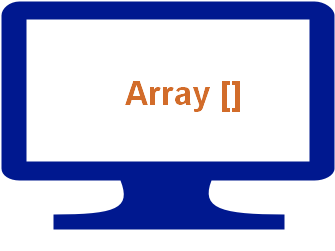# Joining two or more arrays by using concatBy using concat function we can create new array by adding two or more arrays. This function concat does not alter any structure of elements used as arguments for creating the new array.

By using concat we can add elements to an existing array.

Let us start with adding or joining two arrays. We have created two arrays , one is our script array and other one is browsers array. We will join these two arrays by using concat function.

Here is the code
``````var scripts = new Array();
scripts = "PHP";
scripts = "ASP";
scripts = "JavaScript";
scripts = "HTML";

var browsers=new Array();
browsers = "Internet Explorer";
browsers = "FireFox";
browsers = "NetScape";

var both = scripts.concat(browsers);
document.write(both.toString());``````
The output of above code is here
``PHP,ASP,JavaScript,HTML,Internet Explorer,FireFox,NetScape ``
We can use concat to add elements to an existing array. Here is the code.
``````var new_one = scripts.concat("ie","ff","ns");
document.write(new_one.toString());
``````
The output of above code is
``PHP,ASP,JavaScript,HTML,ie,ff,ns``## Subscribe

* indicates required
Subscribe to plus2netplus2net.com

 sophal 25-10-2010 how to write code to defend user input only number in java script?

Post your comments , suggestion , error , requirements etc here